21.2 (EAP/Beta)
21.1
20.2
20.1
19.2
19.1
18.2
18.1
17.2

# Calculate Summaries

The chart control provides built-in and custom summary functions, which can be calculated for data-bound series on the series point values. This document describes summary functions and ways of applying them.

In addition to summary functions, the chart control can automatically aggregate data depending on its size. For more information, refer to Data Aggregation.

The document consists of the following sections.

## Built-in Summary Functions

The chart control provides the following built-in summary functions:

• minimum (“MIN”),
• maximum (“MAX”),
• sum (“SUM”),
• average (“AVERAGE”),
• count (“COUNT”).
##### IMPORTANT

Automatic summary functions can be calculated only for those Series Views, which operate with data points with only 1 value for each. If series data points have 2 or more values (e.g., range, Gantt, Financial and Bubble series views), you should use Custom Summary Functions instead.

The summary function can’t be calculated when the ScaleOptionsBase.ScaleMode is set to Continuous.

The table below demonstrates a chart that utilizes a Point series, with a summary and no summary applied to its data.

Summary function Resulting image
Without summary function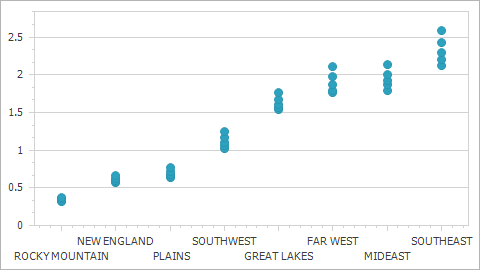Average summary function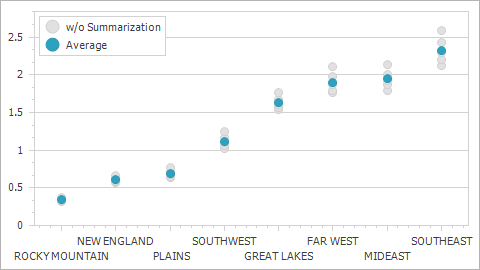Note that the chart control should be aware of the specified measure unit before you apply a summary function to series data. To illustrate this behavior, consider the chart control bound to the following data table.

SeriesPoint Argument Value
 1 5
 1 10
 2 20
 2 40
 3 20
 3 65
 5 15
 6 25
 7 50
 8 25
 9 65
 10 25
 10 15
 20 120
 20 120

The table below shows how the SUM summary function depends on the measure unit.

Property values

Resulting image

MeasureUnit = Ones

SummaryFunction = SUM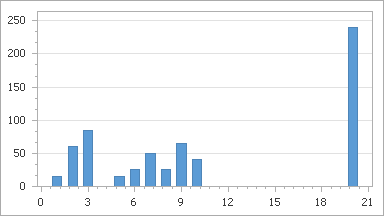MeasureUnit = Tens

SummaryFunction = SUM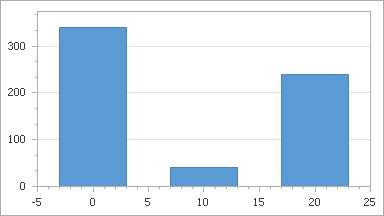In the charts above, the measure unit is specified manually in the manual scale mode (the ScaleOptionsBase.ScaleMode property is set to Manual) using the NumericSummaryOptions.MeasureUnit (DateTimeSummaryOptions.MeasureUnit or TimeSpanSummaryOptions.MeasureUnit) property.

If you need the chart control to calculate a measure unit and apply it to the X-axis automatically depending on the chart data, select the automatic scale mode (the ScaleOptionsBase.ScaleMode property is set to Automatic). For more information, refer to Data Aggregation.

##### NOTE

If you specify the summary function, the aggregate function (ScaleGridOptionsBase.AggregateFunction) can’t be applied to data.

In addition to the built-in summary functions, you can create a custom one to calculate a summary value in any way you wish. For more details, see the Custom Summary Functions section.

## Example

Consider the chart control bound to a data source. For instance, take a look at a chart from Lesson 4 (see the “Create Data Objects and Bind a ChartControl” section), which is bound to the GSP database.

Add a Point series to the chart. Then, set the SeriesBase.ArgumentDataMember property to the Region data field, and SeriesBase.ValueDataMembers property to GSP.

This initial chart is shown below.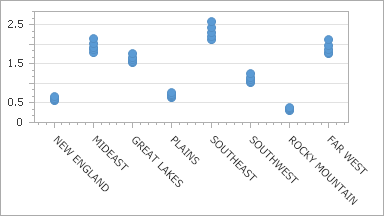To calculate the AVERAGE function for the GSP data field, do the following.

• Select the required series and in the Properties window, locate its SummaryFunction property.
• Click the ellipsis button to invoke the Summary Function dialog. In the invoked dialog, choose the required AVERAGE summary function and the data field to be averaged.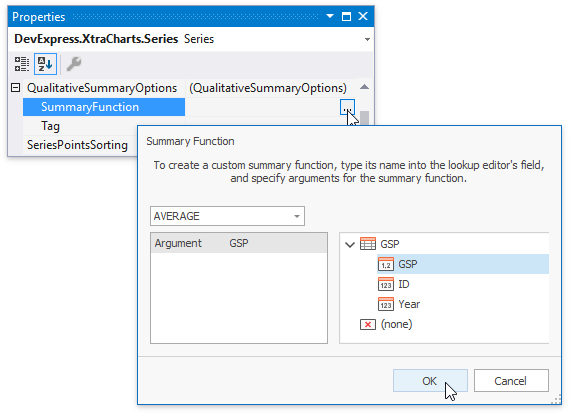• To apply the changes and quit the dialog, click OK.

The result is shown in the image below.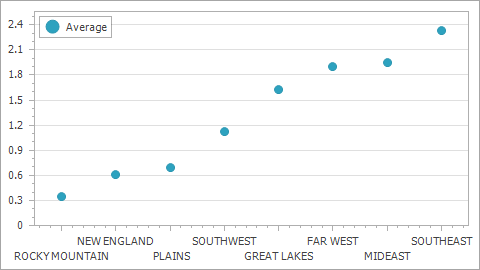The following code snippet illustrates how to do the same at runtime.

``````series1.QualitativeSummaryOptions.SummaryFunction = "AVERAGE([GSP])";
``````
##### NOTE

You need to choose appropriate SummaryOptions (DateTimeSummaryOptions, TimeSpanSummaryOptions, NumericSummaryOptions or QualitativeSummaryOptions) depending on the type of series point arguments.

## Custom Summary Functions

The following example demonstrates how to create a custom summary function, which returns an OHLC point calculated by the passed array of values. To accomplish this task, create a SummaryFunction delegate and register it using the ChartControl.RegisterSummaryFunction method:

``````// Declare the Financial summary function.
private static SeriesPoint[] CalculateProductValue(
Series series,
object argument,
string[] functionArguments,
DataSourceValues[] values,
object[] colors
) {
string functionArgument = functionArguments;
int lastIndex = values.Length - 1;

double open = Convert.ToDouble(values[functionArgument], CultureInfo.InvariantCulture);
double close = Convert.ToDouble(values[lastIndex][functionArgument], CultureInfo.InvariantCulture);
double high = Math.Max(open, close);
double low = Math.Min(open, close);
for (int i = 1; i < lastIndex; i++) {
high = Math.Max(high, Convert.ToDouble(values[i][functionArgument], CultureInfo.InvariantCulture));
low = Math.Min(low, Convert.ToDouble(values[i][functionArgument], CultureInfo.InvariantCulture));
}
// Return the result.
return new SeriesPoint[] {
new SeriesPoint(argument, high, low, open, close)
};
}

private void Form1_Load(object sender, EventArgs e) {
// Register the summary function in a chart.
chartControl.RegisterSummaryFunction(
name: "FINANCIAL",
displayName: "Financial",
resultScaleType: ScaleType.Numerical,
resultDimension: 4,
argumentDescriptions: new SummaryFunctionArgumentDescription[] {
new SummaryFunctionArgumentDescription("Value", ScaleType.Numerical)
},
function: CalculateProductValue
);

Series series = chartControl.Series["EurUsd"];
series.ArgumentDataMember = "DateTime";
// Note that ValueDataMembers are not specified.
series.DateTimeSummaryOptions.SummaryFunction = "FINANCIAL([Value])";
}
``````